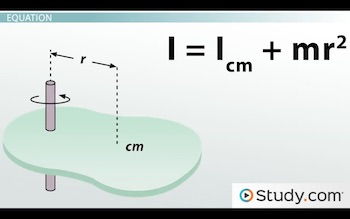# The Parallel-Axis Theorem & the Moment of Inertia

An error occurred trying to load this video.

Try refreshing the page, or contact customer support.

Coming up next: Kinetic Energy of Rotation

### You're on a roll. Keep up the good work!

Replay
Your next lesson will play in 10 seconds
• 0:02 What Is the Parallel…
• 1:36 Equation
• 2:30 Example Calculation
• 4:11 Lesson Summary

Want to watch this again later?

Timeline
Autoplay
Autoplay
Speed

#### Recommended Lessons and Courses for You

Lesson Transcript
Instructor: David Wood

David has taught Honors Physics, AP Physics, IB Physics and general science courses. He has a Masters in Education, and a Bachelors in Physics.

After watching this lesson, you will be able to explain what the parallel axis theorem is and how it is used. You will also be able to use the theorem to solve problems. A short quiz will follow.

## What Is the Parallel Axis Theorem?

In physics, we love to simplify situations. After all, who wants to do complex, calculus-based math and spend hour upon hour playing with algebra? Well, actually, I suppose a lot of physicists do like that. But only when it's genuinely necessary.

In other lessons, we've talked about the moment of inertia. Rotational inertia, otherwise known as moment of inertia, is a number that represents how much mass a rotating object has and how it is distributed. An object with more rotational inertia is harder to accelerate. Moment of inertia is measured in kilogram/meters squared.

But everything we've focused on involves situations that have nice, uniform objects. Spheres, shells, rings... anything symmetrical. And it also assumed that those objects were rotating around an axis that went straight through the center of mass of the object. But what happens when that's not the case?

Well, you could do a load of complex calculus. Or, if you don't like calculus, like most people, you could use the parallel axis theorem.

The parallel axis theorem states that the moment of inertia of an object around a particular axis is equal to the moment of inertia around a parallel axis that goes through the center of mass, plus the mass of the object, multiplied by the distance to that parallel axis, squared.

The moment of inertia around the center of mass is a minimum value. If you move the rotation axis elsewhere, the moment of inertia, how difficult it is to slow or speed up the rotation of the object, increases.

## Equation

The parallel axis theorem is much easier to understand in equation form than in words. Here it is:

I = Icm + mr ^2

This diagram shows a randomly shaped object with a rotation axis that doesn't go through the center of mass:But if you take a parallel rotation axis that does go through the center of mass, we can use that to figure out the moment of inertia through the actual rotation axis.

If we know, or we can figure out, the moment of inertia through the center of mass axis, Icm, measured in kilogram/meters squared, and we know the total mass of the object, m, measured in kilograms, and the distance the parallel axis is away from the center of mass, r, measured in meters, we can just plug those numbers in and figure out the moment of inertia through our off-center rotational axis.

So, that's probably a little easier to understand now. But you know what would make it even better? An example.

## Example Calculation

One day you're cleaning the basement when you find a really old bedroom pillow. One of those super lumpy pillows that should have been thrown out years ago. Before you throw it out, being an amateur physicist, you decide to do an experiment on it. You unceremoniously grab a knitting needle from your grandma, stab it through the middle, and spin the pillow around the needle.

To unlock this lesson you must be a Study.com Member.

### Register to view this lesson

Are you a student or a teacher?

### Unlock Your Education

#### See for yourself why 30 million people use Study.com

##### Become a Study.com member and start learning now.
Back
What teachers are saying about Study.com

### Earning College Credit

Did you know… We have over 160 college courses that prepare you to earn credit by exam that is accepted by over 1,500 colleges and universities. You can test out of the first two years of college and save thousands off your degree. Anyone can earn credit-by-exam regardless of age or education level.